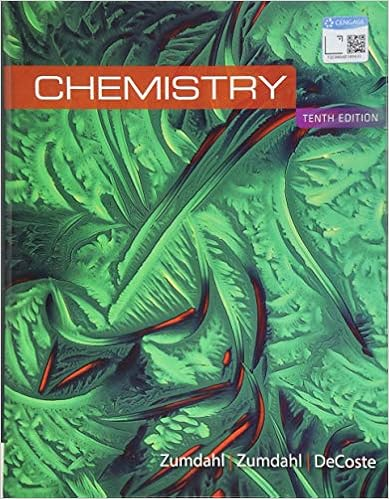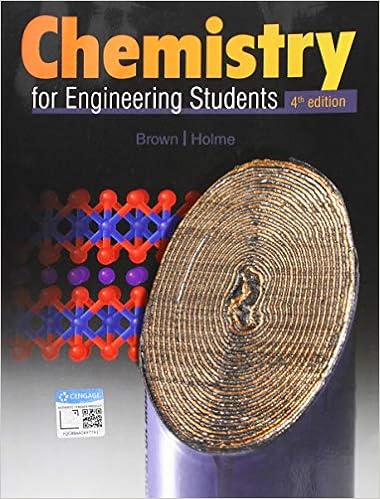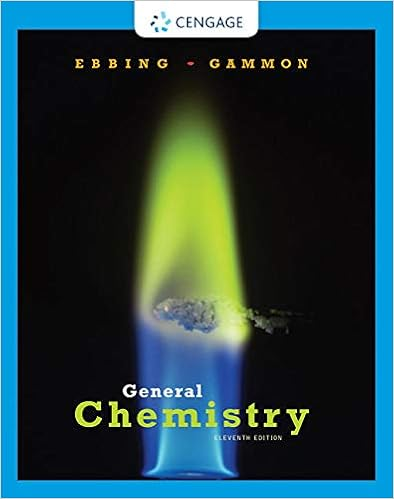# 2 g h 1989 kj o 3 g 15o 2 g h 1423 kj o 2 g 2o g h

• Test Prep
• 19
• 100% (1) 1 out of 1 people found this document helpful

This preview shows page 12 - 17 out of 19 pages.

2(g)H= –198.9 kJO3(g)1.5O2(g)H= –142.3 kJO2(g)2O(g)H= 495.0 kJA.–551.6 kJB.153.8 kJC.190.9 kJD.–304.1 kJE.438.4 kJ
____38.Which one of the following sets of quantum numbers isnotpermitted??
____39.What is the electronic configuration for Cr+?4d3
##### We have textbook solutions for you!The document you are viewing contains questions related to this textbook.
Chapter 16 / Exercise 50
Chemistry: The Molecular Science
Moore/StanitskiExpert VerifiedBrowse all Textbook Solutions
____40.Which of the following species has the smallest radius?
____41.Select the element with the highest first ionization energy.A.MgB.CaC.BaD.RaE.K
____42.From the data given below, calculate the approximate enthalpy change of reaction for the reactionbelow.CH4(g) + 2O2(g)CO2(g) + 2H2O(g)bond enthalpykJ/molC–H414C–C347C=C611C–O351C=O803O–H463O=O498H–H436
Form A, Page 13 of 17
____43.Which statement best describes the formal charges on the atoms inPO43?
____44.Construct correct Lewis dot structures for the three molecules below. Determine whichcompounds, if any, have an extended octet.CF4SF4ClF3IIIIII
____45.Using VSEPR Theory, predict the electron-pair geometry, the molecular geometry, and thesmallest approximate F–Cl–F bond angle of ClF3.A.trigonal bipyramidal, T-shaped, 90B.tetrahedral, trigonal planar, 109.5C.trigonal bipyramidal, trigonal pyramidal, 109.5D.trigonal bipyramidal, trigonal planar, 120E.trigonal planar, trigonal planar, 120
Form A, Page 14 of 17
____46.Which of the following has a trigonal planar molecular geometry?I.nitrate ionII.carbonate ionIII.sulfite ionIV.selenite ion
____47.Which of the following molecules are polar?I.ICl4II.NH4+III.IF5IV. RnF2
____48.Determine the hybridization around each central atom.NH2NH3CO32–23d
Form A, Page 15 of 17
____49.Which of the following molecules are paramagnetic?B2, C2, N2, O2, F2A.B2and N2B.B2and O2C.N2and O2D.N2and F2E.C2, O2, and F2
____50.What is the bond order for NO?
Form A, Page 16 of 17____51.***Calculate the lattice energy of magnesium sulfide from the data given below:***Mg(s)Mg(g)H° = 148 kJ/molMg(g)Mg2+(g)+2eH° = 2186 kJ/molS8(s)8S(g)H° = 2232 kJ/molS(g)+2eS2–(g)H° = 450 kJ/mol8Mg(s) +S8(s)8MgS(s)H° = –2744 kJ/molMg2+(g) + S2–(g)MgS(s)H°lattice= ?

Course Hero member to access this document

Course Hero member to access this document

End of preview. Want to read all 19 pages?

Course Hero member to access this document

Term
Spring
Professor
Kerber
Tags
##### We have textbook solutions for you!
The document you are viewing contains questions related to this textbook.The document you are viewing contains questions related to this textbook.
Chapter 16 / Exercise 50
Chemistry: The Molecular Science
Moore/StanitskiExpert Verified
•••